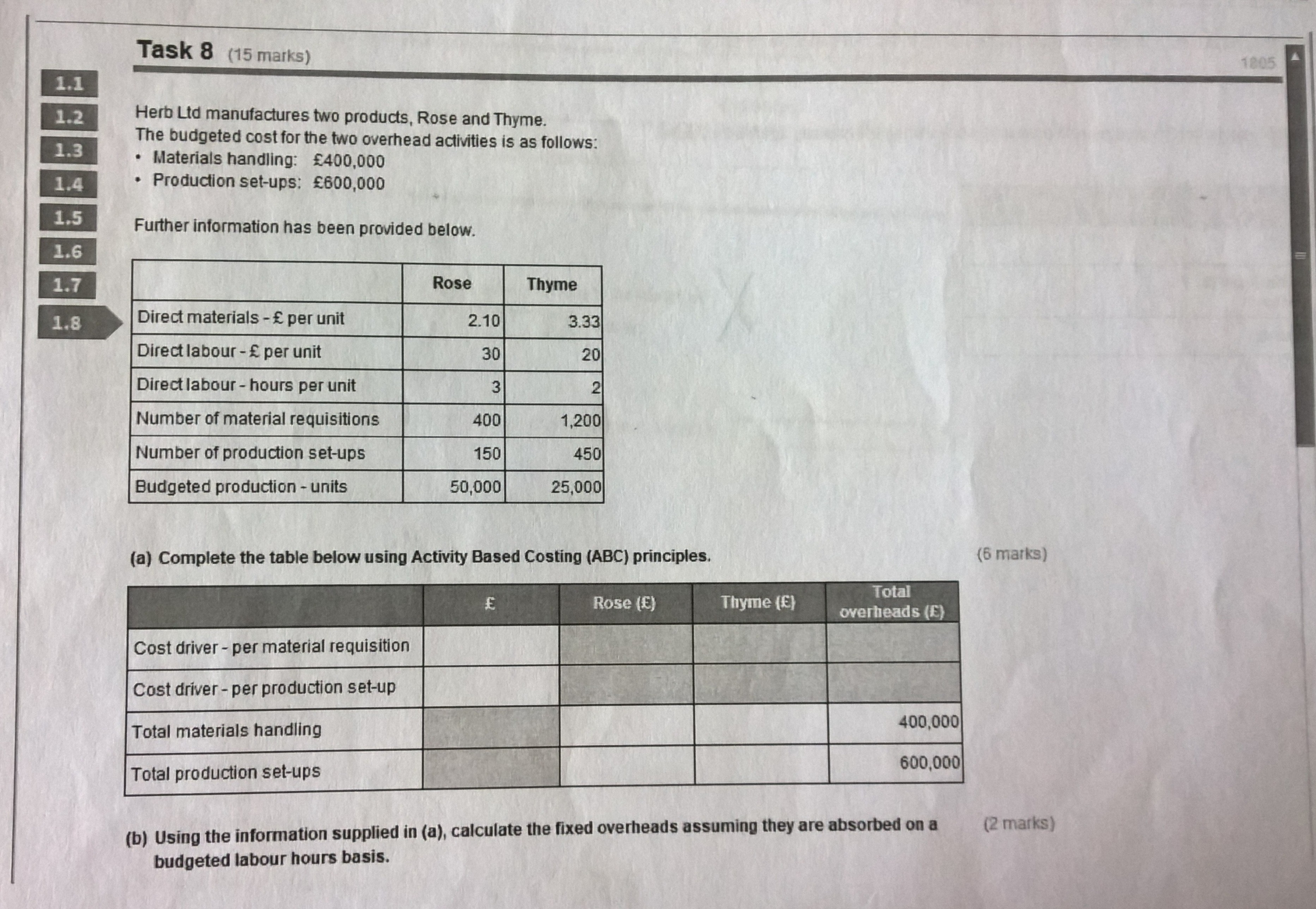Current updates regarding coronavirus (Covid-19) and the precautions AAT are taking will be continually updated on the below page.

We hope you are all safe and well and if you need us we will be here. 💚

# Practice assessment 1 - Task 8a

Hi,

Any help would be much appreciated! Thanks!• can anyone explain how to get the answers on this one please
• Cost material per mat requisition is £400000/1600(400+1200)=£250
Cost material per set up 600000/600(150+450)=£1000
AAT Level 2. 2018 Distinction
AAT Level 3. 2018 Distinction
AAT Level 4. 2019 FSLC 100% MABU 93% MADC 82% PDSY 80%
• Following from the previous post the total materials handling for Rose is £250x400 requisitions=100000 and for Thyme £250x1200=300000
The set up is 1000*150=150000 for Rose and Tyme 1000*450=450000
AAT Level 2. 2018 Distinction
AAT Level 3. 2018 Distinction
AAT Level 4. 2019 FSLC 100% MABU 93% MADC 82% PDSY 80%
• Registered, MAAT, AATQB, AAT Licensed Accountant Posts: 116
Ok, so you already know that the total materials handling is 400,000 and 600,000!

For the cost driver per material requisition, it is: 400,000 / (400+1200) = 250
For the cost driver per production set up it is: 600,000 / (150+450) = 1000

Total material handling for Rose will be: 400 x 250 = 100,000
Total material handling for Thyme will be: 1200 x 250 = 300,000

Total production set up for Rose will be 150 x 1000 = 150,000
Total production set up for Thyme will be: 450 x 1000 = 450,000

Hope that helps.
• Registered, MAAT, AATQB, AAT Licensed Accountant Posts: 116
If you are stuck on b), then you are told that Rose takes 3 hours to complete a unit and Thyme takes 2 hours to complete a unit. Therefore,
For Rose it will be 3 x 50,000 = 150,000
For Thyme it will be 2 x 25,000 = 50,000
Total is 200,000

Then:work out the hourly rate. So that would be 400,000 + 600,000 / 200,000 = £5 per hour

Therefore, for Rose, the calculation will be 150,000 x £5 = £750,000
And for Thyme, the calculation will be 50,000 x £5 = 250,000
• Registered, MAAT, AATQB, AAT Licensed Accountant Posts: 116
You are very welcome.